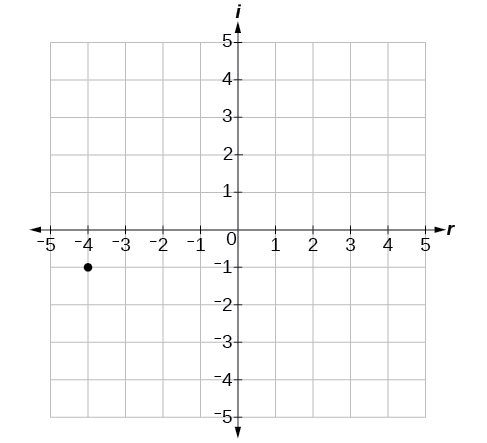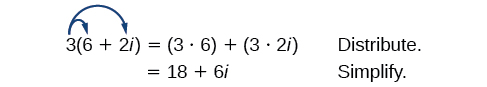# 2.4 Complex numbers  (Page 2/8)

 Page 2 / 8

Let’s consider the number $\text{\hspace{0.17em}}-2+3i.\text{\hspace{0.17em}}$ The real part of the complex number is $\text{\hspace{0.17em}}-2\text{\hspace{0.17em}}$ and the imaginary part is 3. We plot the ordered pair $\text{\hspace{0.17em}}\left(-2,3\right)\text{\hspace{0.17em}}$ to represent the complex number $\text{\hspace{0.17em}}-2+3i,$ as shown in [link] .

## Complex plane

In the complex plane, the horizontal axis is the real axis, and the vertical axis is the imaginary axis, as shown in [link] .

Given a complex number, represent its components on the complex plane.

1. Determine the real part and the imaginary part of the complex number.
2. Move along the horizontal axis to show the real part of the number.
3. Move parallel to the vertical axis to show the imaginary part of the number.
4. Plot the point.

## Plotting a complex number on the complex plane

Plot the complex number $\text{\hspace{0.17em}}3-4i\text{\hspace{0.17em}}$ on the complex plane.

The real part of the complex number is $\text{\hspace{0.17em}}3,$ and the imaginary part is –4. We plot the ordered pair $\text{\hspace{0.17em}}\left(3,-4\right)\text{\hspace{0.17em}}$ as shown in [link] .

Plot the complex number $\text{\hspace{0.17em}}-4-i\text{\hspace{0.17em}}$ on the complex plane.## Adding and subtracting complex numbers

Just as with real numbers, we can perform arithmetic operations on complex numbers. To add or subtract complex numbers, we combine the real parts and then combine the imaginary parts.

## Complex numbers: addition and subtraction

$\left(a+bi\right)+\left(c+di\right)=\left(a+c\right)+\left(b+d\right)i$

Subtracting complex numbers:

$\left(a+bi\right)-\left(c+di\right)=\left(a-c\right)+\left(b-d\right)i$

Given two complex numbers, find the sum or difference.

1. Identify the real and imaginary parts of each number.
2. Add or subtract the real parts.
3. Add or subtract the imaginary parts.

## Adding and subtracting complex numbers

1. $\left(3-4i\right)+\left(2+5i\right)$
2. $\left(-5+7i\right)-\left(-11+2i\right)$

1. $\begin{array}{ccc}\hfill \left(3-4i\right)+\left(2+5i\right)& =& 3-4i+2+5i\hfill \\ & =& 3+2+\left(-4i\right)+5i\hfill \\ & =& \left(3+2\right)+\left(-4+5\right)i\hfill \\ & =& 5+i\hfill \end{array}$

2. $\begin{array}{ccc}\hfill \left(-5+7i\right)-\left(-11+2i\right)& =& -5+7i+11-2i\hfill \\ & =& -5+11+7i-2i\hfill \\ & =& \left(-5+11\right)+\left(7-2\right)i\hfill \\ & =& 6+5i\hfill \end{array}$

Subtract $\text{\hspace{0.17em}}2+5i\text{\hspace{0.17em}}$ from $\text{\hspace{0.17em}}3–4i.$

$\left(3-4i\right)-\left(2+5i\right)=1-9i$

## Multiplying complex numbers

Multiplying complex numbers is much like multiplying binomials. The major difference is that we work with the real and imaginary parts separately.

## Multiplying a complex number by a real number

Lets begin by multiplying a complex number by a real number. We distribute the real number just as we would with a binomial. Consider, for example, $\text{\hspace{0.17em}}3\left(6+2i\right)$ :Given a complex number and a real number, multiply to find the product.

1. Use the distributive property.
2. Simplify.

## Multiplying a complex number by a real number

Find the product $\text{\hspace{0.17em}}4\left(2+5i\right).$

Distribute the 4.

$\begin{array}{ccc}\hfill 4\left(2+5i\right)& =& \left(4\cdot 2\right)+\left(4\cdot 5i\right)\hfill \\ & =& 8+20i\hfill \end{array}$

Find the product: $\text{\hspace{0.17em}}\frac{1}{2}\left(5-2i\right).$

$\frac{5}{2}-i$

## Multiplying complex numbers together

Now, let’s multiply two complex numbers. We can use either the distributive property or more specifically the FOIL method because we are dealing with binomials. Recall that FOIL is an acronym for multiplying First, Inner, Outer, and Last terms together. The difference with complex numbers is that when we get a squared term, $\text{\hspace{0.17em}}{i}^{2},$ it equals $\text{\hspace{0.17em}}-1.$

Given two complex numbers, multiply to find the product.

1. Use the distributive property or the FOIL method.
2. Remember that $\text{\hspace{0.17em}}{i}^{2}=-1.$
3. Group together the real terms and the imaginary terms

A laser rangefinder is locked on a comet approaching Earth. The distance g(x), in kilometers, of the comet after x days, for x in the interval 0 to 30 days, is given by g(x)=250,000csc(π30x). Graph g(x) on the interval [0, 35]. Evaluate g(5)  and interpret the information. What is the minimum distance between the comet and Earth? When does this occur? To which constant in the equation does this correspond? Find and discuss the meaning of any vertical asymptotes.
The sequence is {1,-1,1-1.....} has
how can we solve this problem
Sin(A+B) = sinBcosA+cosBsinA
Prove it
Eseka
Eseka
hi
Joel
June needs 45 gallons of punch. 2 different coolers. Bigger cooler is 5 times as large as smaller cooler. How many gallons in each cooler?
7.5 and 37.5
Nando
find the sum of 28th term of the AP 3+10+17+---------
I think you should say "28 terms" instead of "28th term"
Vedant
the 28th term is 175
Nando
192
Kenneth
if sequence sn is a such that sn>0 for all n and lim sn=0than prove that lim (s1 s2............ sn) ke hole power n =n
write down the polynomial function with root 1/3,2,-3 with solution
if A and B are subspaces of V prove that (A+B)/B=A/(A-B)
write down the value of each of the following in surd form a)cos(-65°) b)sin(-180°)c)tan(225°)d)tan(135°)
Prove that (sinA/1-cosA - 1-cosA/sinA) (cosA/1-sinA - 1-sinA/cosA) = 4
what is the answer to dividing negative index
In a triangle ABC prove that. (b+c)cosA+(c+a)cosB+(a+b)cisC=a+b+c.
give me the waec 2019 questionsByByByByByByBy